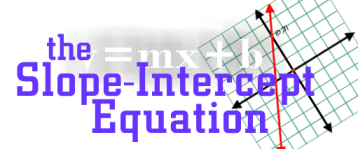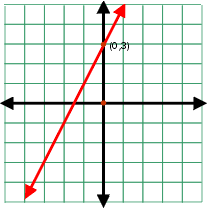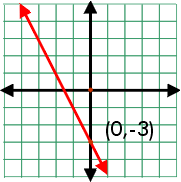The slope intercept form of a line is y = mx + b. Where m is the slope and b is the y-intercept. The y-intercept is the point where the line crosses the y-axis. In the example to the right, the line crosses the y-axis at point (0,3). Therefore b = 3. The slope is 2.The slope intercept equation is y = 2x + 3.Write the slope intercept equation for this line. The slope is -2 and b=-3.